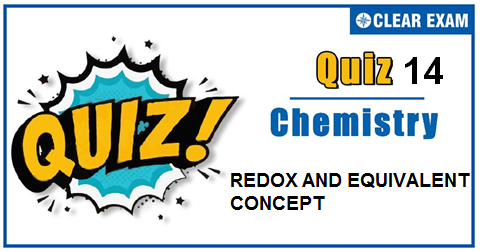## REDOX AND EQUIVALENT CONCEPT QUIZ-14

The one subject in NEET which is candidates who can easily attain good marks is Chemistry. That's the reason, often, one doesn't pay notice and choose to compromise it. But if one wants to rank above others, the tip is to be thorough with NEET chemistry concepts. The understanding of reactions and definite basic understanding is what requires major attention in Chemistry but once done it only gets simpler from there. The main focus on the to-do list should be on getting a hang of the NCERT syllabus of NEET chemistry. .

Q1. Millimole of a solute in a solution can be given by :
•  M × V(in litre)
•  M × V(in mL)
•  (wt.)/(mol.wt.) × 1000
•  Both (b) and (c)
Solution
Both are same

Q2.Which can act as oxidant?
•  H2 O2
•  H2 S
•  NH3
•  None of these
Solution
Oxygen of H2 O2 gets reduced from − 1 to −2.

Q3.  When an acidified solution of ferrous ammonium sulphate is treated with KMnO4 solution, the ion which is oxidised is :
•   Fe(2+)
•  SO4(2-)
•  NH4+
•  MnO4-
Solution
Fe(2+)⟶Fe(3+)+e.

Q4. In which reaction the underlined substance has been reduced?
•  Carbon monoxide + copper oxide ⟶ carbon dioxide + copper
•  Copper oxide + hydrochloric acid ⟶ water + copper chloride
•  Steam + iron ⟶ hydrogen + iron oxide
•  Hydrogen + iron oxide ⟶ water + iron
Solution
H2(1+) O ⟶H20 ; Steam is reduced.

Q5.Sulphurous acid can be used as :
•  Oxidising agent
•  Reducing agent
•  Bleaching agent
•  All of these
Solution
S in H2 SO3 is in +4 oxidation state. It lies in between its maximum and minimum oxidation state, i.e,+6 and -2 and thus, S can increase or decrease its ox.no.as the case may be.

Q6. Oxalic acid on reacting with acidified KMnO4 is oxidised to :
•  CO and H2
•  CO2and H2
• CO2 and H2 O
•  CO and H2O
Solution
Mn(7+)+5e ⟶Mn(2+) C2(3+) ⟶2C(4+)+2e

Q7.The oxidation number of Xe in XeF4 and XeO2 is
•  +6
•  +4
•  +1
•  +3
Solution
The oxidation state of Xe in both XeO2 and XeF4 is 4. XeO2 XeF4 x+2(-2)=0 x+4(-1)=0 x=4 x=4

Q8.When NaCl is dissolved in water, the sodium ion becomes :
•  Oxidized
•  TReduced
•  Hydrolsed
•  hydrated
Solution
Ions are hydrated on dissolution of salt in water.

Q9.Which change requires a reducing agent?
•  CrO4(2-) ⟶CrO7(2-)
•  BrO3- ⟶BrO-
•  H2 O2 ⟶O2
•  Al(OH)3 ⟶Al(OH)4-
Solution
4e+Br(5+)⟶Br(1+) ; Thus, BrO3- is to be reduced by a reducing agent.

Q10. Oxidation state of oxygen is −1 in the compound :
•  NO2
•  MnO2
•  PbO2
• Na2 O2
Solution
Na2 O2 is sodium peroxide.## Want to know more

Please fill in the details below:

## Latest NEET Articles\$type=three\$c=3\$author=hide\$comment=hide\$rm=hide\$date=hide\$snippet=hide

Name

ltr
item
BEST NEET COACHING CENTER | BEST IIT JEE COACHING INSTITUTE | BEST NEET & IIT JEE COACHING: REDOX AND EQUIVALENT CONCEPT QUIZ-14
REDOX AND EQUIVALENT CONCEPT QUIZ-14